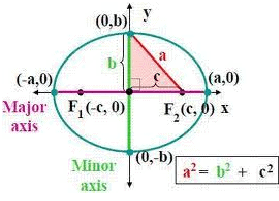Courses

# Notes | EduRev

## JEE: Notes | EduRev

The document Notes | EduRev is a part of the JEE Course Mock Test Series for JEE Main & Advanced 2022.
All you need of JEE at this link: JEE
• The standard equation of ellipse with reference to its principal axis along the coordinate axis is given by x2/a+ y2/b2 = 1
• In the standard equation, a >b and b2 = a2 (1-e2) Hence, the relation between a and b is a2 – b2 = a2e2, where ‘e’ is the eccentricity and 0 < e < 1.
• The foci of the ellipse are S(ae, 0) and S’ = (-ae, 0)• Equations of the directrices are given by x = a/e and x = -a/e
• The coordinates of vertices are A’ = (-a, 0) and A = (a,0)
• The lengths of the major and minor axis are 2a and 2b respectively.
• The length of latus rectum is 2b2/a = 2a(1-e2)
• The sum of the focal distances of any pint on the ellipse is equal to the major axis. As a result, the distance of focus from the extremity of a minor axis is equal to semi major axis.
• If a question does not mention the relation between a and b then by convention a is assumed to be greater than b i.e. a > b.
• The point P(x1, y1) lies outside, inside or on the ellipse according as x12/a2 + y12/b– 1>< or = 0.
• In parametric form, the equations x = a cos θ and y = b sin θ together represent the ellipse.
• The line y = mx + c meets the ellipse x2/a2 + y2/b2 = 1 in either two real, coincident or imaginary points according to whether c2 is < = or > a2m+ b2
• The equation y = mx + c is a tangent to the ellipse if c2 = a2m2+ b2
• The equation of the chord of ellipse that joins two points with eccentric angles α and β is given byx/acos (α + β)/2 + y/b sin (α + β)/2 = cos (α - β)/2
• The equation of tangent to the ellipse at the point (x1, y1) is given byxx1/a2 + yy1/b2 = 1
• In parametric form, (xcosθ) /a + (ysinθ/b) is the tangent to the ellipse at the point (a cos θ a, b sin θ)
• Equation of normal
1. Equation of normal at the point (x1,y1) is
a2x/x1 – b2y/y1 = a2- b2 = a2e2
2.Equation of normal at the point (a cos θ a, b sin θ) is ax secθ – by cosec θ = (a2-b2)
3. Equation of normal in terms of its slope ‘m’ is
y = mx – [(a2-b2)m /√a2+b2m2]
• The equation of director circle is x2+ y2= a2 + b
• The portion of the tangent to an ellipse between the point of contact and the directrix subtends a right angle at the corresponding focus.
The perpendiculars from the center upon all chords which join the ends of any particular diameters of the ellipse are of constant length.
The document Notes | EduRev is a part of the JEE Course Mock Test Series for JEE Main & Advanced 2022.
All you need of JEE at this link: JEEUse Code STAYHOME200 and get INR 200 additional OFF Use Coupon Code
All Tests, Videos & Notes of JEE: JEE

### Top Courses for JEE## Mock Test Series for JEE Main & Advanced 2022

2 videos|258 docs|160 tests

### Top Courses for JEETrack your progress, build streaks, highlight & save important lessons and more!

,

,

,

,

,

,

,

,

,

,

,

,

,

,

,

,

,

,

,

,

,

;